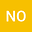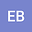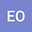Analysis of a fractional mathematical model for Zika virus under the framework of singular and nonsingular kernels
•••• Newton Okposo,
• Ebenzer Bonyah,
• Emamuzo Okposo
Newton Okposo
Delta State University

Corresponding Author:newstar4sure@gmail.com

Author ProfileEbenzer Bonyah
Kumasi Technical University
Author ProfileEmamuzo Okposo
Delta State College of Education
Author Profile## Abstract

In this paper, we investigate the dynamics of a fractional zika virus model (ZIKV) with Caputo, Caputo-Fabrizio-Caputo (CFC) and Atangana-Baleanu-Caputo (ABC) derivatives. Firstly the basic properties of the classical integer order model are furnished followed by the equilibrium points and basic reproduction number. Furthermore, with respect to the Caputo, CFC and ABC derivatives, we establish via a fixed point technique that under certain conditions the fractional ZIKV model admits a unique system of solutions. The Adams-Bashforth numerical scheme incorporating the fractional order parameter is then used to obtain numerical schemes for the approximate solutions of the fractional ZIKV model with respect to each of the considered fractional differential operators. Finally, with a view to visualize the behaviour of the approximate solutions to fractional ZIKV model with respect to each of the fractional differential operators, we do some numerical simulations for distinct values of the fractional order parameter.